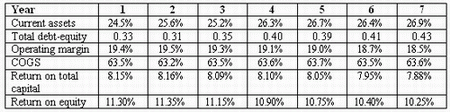### CFA Practice Question

The following table presents selected common size income statement and balance sheet data for Curative Drugs.If Curative had total debt of \$34.5 million outstanding in year 4, what was the interest paid in that year?
A. \$0.38 million
B. \$3.80 million
C. \$2.54 million
Explanation: This requires manipulation of several ratios. In year 4:

Total-debt to equity = 0.40 = TD/Equity => Equity = 34.5/0.40 = 86.25 million
ROE = NI/Equity = 0.109 => NI = 0.109 x 86.25 = 9.40 million
Return on total capital = (NI + Interest) / (Total debt + Equity) = 0.081 => Interest = 0.081 x (34.5 + 86.25) - 9.40 = 0.38 million

User Comment
uberstyle Fantastic question. I just hope they don't ask any like it. Know your ratios!
dealsoutlook damn..didnt know the ratio for ROTC :(
surob Hard question
Nicholas I too commend the AN team for this one. The RoTC got me as well.
sambra yes, but RoTC = ebit/TC, so taxes are missing here : (Ni + Int)*(1-taxes)^-1/ total capital
grezavi Doesn't EBIT stands for Earnings before Int Exp & taxes anyways, ROTC=EBIT/TC is a quicker way than indicated by sambra above.
11Blaise pretty excited to post my first comment
ROTC-ROE = Interest
Interest = 9,780,750-9,400,125= 380,000.
chingg2 i would have just guessed and skipped this question to save time
copus Agree with chingg2 - i would have taken a flyer on this one and moved onto the next question.
CJI19 \$.38mm only one that makes sense - \$3.8mm on \$34.5mm in debt would be 11%+ - No firm has a higher cost of debt than equity
birdperson @cji19 -- i think that 2.54 could make sense...
janis36 Unreal tournament. I don't believe I can do this in 90 sec ever.
Rsanches good question
ashish100 AN isn't supposed be exactly like the exams you bums. They're just trying to help you be extra prepared and pass the exam god forbid.

Also if you are that good, it is POSSIBLE to do it in 90 secs.
mikus Outstanding question!
will080912 That question was a kille
atlootah what a question
pigletin i refuse to take time to remember these formula